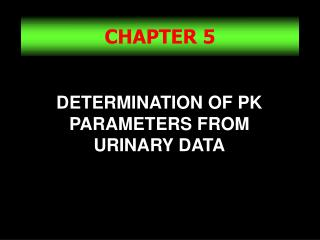DownloadDownload PresentationCHAPTER 5

# CHAPTER 5

Download Presentation## CHAPTER 5

- - - - - - - - - - - - - - - - - - - - - - - - - - - E N D - - - - - - - - - - - - - - - - - - - - - - - - - - -
##### Presentation Transcript

1. CHAPTER 5 DETERMINATION OF PK PARAMETERS FROM URINARY DATA

2. Calculation of kel from Urinary Excretion Data after I.V. Injection kel can be calculated from urinary excretion data. The excretion rate of the drug is assumed to be first order. ke is the renal excretion rate constant. Du is the amount of drug excreted unchanged in the urine.

3. Elimination kel = ke + km

4. Scheme of the Model DB= CpVd ke IV Dose Du For a single i.v. dose, km dDu/dt = keDB

5. dDu = keDB Rate of Drug Excretion in the Urine dt Equations ButDB= DB0e-kelt Therefore,

6. keDB Slope= -kel dDu/dt Time Plotting on a Semilog Paper Plot dDu/dt vs. Time

7. Example

8. Difference between t and t* t is the time interval for collection of urine sample. t* is the midpoint of collection period. Assuming renal clearance is constant, Du/t is proportional to plasma drug conc, and plotting Du/t vs. t* is like plotting Cp vs. time. The measured urinary excretion rate reflects the average plasma concentration during the collection interval.

9. Why t* ? Because the drug urinary excretion rate (dDu/dt) cannot be determined experimentally at any given instant. In practice, urine is collected over a specified time interval, and the urine specimen is analyzed for drug. An average urinary excretion rate is then calculated for that collection period. The average dDu/dt is then plotted against the average time (t*).

10. Determination of the non-renal rate constant (knr) knr= is the elimination rate constant for any route of elimination other than renal excretion. kel - ke = knr Since drug elimination occurs mainlythrough renal excretion and metabolism, knr km kel = ke + km

11. Determination of renal clearance Renal clearance, ClR, is defined as the volume of plasma that is cleared of drug per unit of time through the kidney

12. Sigma-Minus Method Also called the Amount of Drug Remaining to be Excreted Method. It is an alternative method for the calculation of kel from urinary excretion data. It is more accurate than the previous method. ke/kel is the fraction of drug excreted unchanged in the urine. (ke/kel)*Dose= total amount of drug excreted unchanged in the urine.

13. Sigma-Minus Method (cont) Equations Where, Du is the cumulative amount of drug excreted unchanged in the urine until time t. (1- e-kelt) is the fraction of drug lost from the body.

14. Sigma-Minus Method (cont) The amount of drug that is ultimately excreted at time infinity will be equal to Du Du = ke/kel (D0) (2) By substituting in the previous equation (1) Du - Du = Du e-kelt (3) To obtain a linear equation: Ln (Du - Du) = ln Du - kelt (4) Where, (Du - Du) is the amount of drug remaining to be excreted.

15. Du Slope= -kel Du-Du Time Sigma-Minus Plot On a semilog paper:

16. Example Use these data to calculate kel

17. Cumulative Amount of Drug Excreted in the Urine One needs to collect urine samples for a minimum of 7-10 half-lives of the drug to assure all the drug is excreted into the urine. Du Plot Cumulative amount excreted Time

18. Renal clearance Renal clearance can be determined from model independent equation

19. Fraction of drug excreted The fraction of drug excreted unchanged in the urine (fe) can be calculated as follows:

20. Comparison between the Rate and the Sigma-Minus Method 1- In the rate method, Du need not be known, and the loss of one urine specimen does not invalidate the entire study. 2- The sigma-minus method needs accurate determination of Du which requires urine collection until drug excretion is complete. 3- Fluctuations in the rate of drug elimination and experimental errors (such as incomplete bladder emptying) cause considerable departure from linearity in the rate method.

21. Comparison (cont) 4- The sigma-minus is less affected by fluctuations in the rate of drug elimination. 5- The rate method is applicable to zero-order elimination process, while sigma-minus method is not. 6- The ke can be obtained from the rate method but not from the sigma-minus method.

22. Problems in Obtaining Valid Urinary Excretion Data 1- A significant fraction of unchanged drug must be excreted in the urine( at least 20 % ). 2- The assay technique must be specific. 3- Frequent sampling is necessary for a good curve description. 4- Urine samples should be collected until almost all drug is excreted(7 t half) 5- Variation in urinary pH and volume cause significant variation in urinary excretion rates. 6- Subjects should be instructed to the importance of complete bladder emptying.